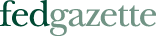A widget example of the Herfindahl-Hirschman Index

Published January 1, 2000  | January 2000 issue

The Herfindahl-Hirschman Index, or HHI, is calculated by squaring the market share of each company in an industry, and then adding the squared values together. For example, assume for a minute that the market in widgets is equally shared by 10 widget makers. Their premerger HHI would be:

102 + 102 + 102 + 102 + 102 + 102 + 102 + 102 + 102 + 102 = 1,000

The highest possible HHI is 10,000 (a monopoly = 100 percent); on the low end, an HHI can be extremely small, of course, because the index declines with each added player and there is, conceivably, no limit to the number that can be added.

Now suppose a merger occurred between two of them, doubling the market share of one company, and shrinking the total market to nine companies. In evaluating mergers, the U.S. Department of Justice (DOJ) looks at the post-merger HHI values, which in the case of the widget makers would be:

202 + (102 + 102 + 102 + 102 + 102 + 102 + 102 + 102) = 400 + 800 = 1,200.

The DOJ also considers any increase in the HHI after the merger, which in this case is 200. In determining whether a merger will affect competition, the DOJ considers a HHI value under 1,000 to be unconcentrated, and mergers within such a market are not considered to have adverse effects on concentration.

An HHI value of 1,000 to 1,800 is considered moderately concentrated, and increases of more than 100 points (like in the case of the widget makers) "raise significant competitive concerns," according to DOJ guidelines.

An HHI value of more than 1,800 is considered highly concentrated, and a post-merger increase of more than 50 points raises red flags with the DOJ. Using the HHI, any four-firm market share of 70 percent would fall in the moderate to highly concentrated category, depending on the distribution of individual market shares.

Top